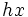# Tour:Left and right coset spaces are naturally isomorphic

PREVIOUS: Right coset of a subgroup| UP: Introduction three (beginners)| NEXT: Index of a subgroup
General instructions for the tour | Pedagogical notes for the tour | Pedagogical notes for this part
PREREQUISITES: Definition of group, subgroup, left coset, right coset, and the fact that inverse map is involutive.
WHAT YOU NEED TO DO: Read and understand the proof below. Fill in any details you think are missing.

## Statement

### Statement with symbols

Let$G$ be a group and$H$ a subgroup of$G$. Then, if$L$ denotes the set of left cosets of$H$ (viz, sets of the form$xH$), and$R$ denotes the set of right cosets of$H$ (viz, sets of the form$Hx$), then there is a natural bijection between$L$ and$R$.

This bijection is induced by the set-theoretic map sending$x$ to$x^{-1}$.

## Facts used

• Inverse map is involutive: we use the fact that$(ab)^{-1} = b^{-1}a^{-1}$, and also that$(a^{-1})^{-1} = a$.

## Proof

Given: A group$G$, subgroup$H$

To prove: The map$x \mapsto x^{-1}$ establishes a bijection between the left cosets of$H$ in$G$ and the right cosets of$H$ in$G$.

Proof: Note that by fact (1), the map$x \mapsto x^{-1}$ is bijective, so every element occurs as the image of exactly one element.

1. The map sends each left coset bijectively to exactly one right coset, and every right coset occurs as the image of exactly one left coset: Any element of the form$xh$ with$h$ in$H$ gets mapped to$h^{-1}x^{-1}$ (we're using fact (1) here), which lies in$Hx^{-1}$. Hence, the image of$xH$ under the inverse map is inside$Hx^{-1}$. Further, any element in$Hx^{-1}$ arises as the image of a unique element under the inverse map and that element is in$xH$: if$hx^{-1}$ is such an element, it arises as the image of the element$xh^{-1} \in xH$ (again, using fact (1)).
2. The map sends each right coset bijectively to exactly one left coset, and every left coset occurs as the image of exactly one right coset: Any element of the form$hx$ with$h$ in$H$ gets mapped to$x^{-1}h^{-1}$, which lies in$x^{-1}H$ (we're using fact (1) here). Hence, the image of$Hx$ under the inverse map is inside$x^{-1}H$. Further, any element in$x^{-1}H$ arises as the image of a unique element under the inverse map under the inverse map, and that element is in$Hx$: if$x^{-1}h$ is such an element, it arises as the image of the element$h^{-1}x \in Hx$ (again using fact (1)).

Thus, the map$x \mapsto x^{-1}$ gives a well-defined bijective map from left cosets to right cosets, as well as from right cosets to left cosets.

WHAT'S MORE: Some further facts about left and right cosets, and some more general remarks about the relation between left and right. Ignore if confusing.

## Related facts

### More general equivalence of left and right

This result is part of a more general result, which states that left and right play equivalent roles in the theory of groups:

### Breakdown for other algebraic structures

The result has no analogue for monoids, semigroups, and other structures. It is true for involutive monoids (monoids equipped with an involution) when the submonoid is closed under that involution. Of course, in the case of monoids, other facts about cosets break down -- for instance, the cosets are not necessarily pairwise disjoint, and they do not necessarily have equal size.